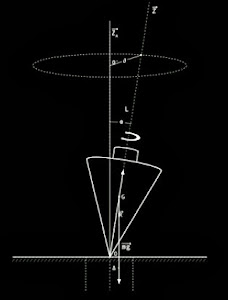### The beauty of mathematics: the spinning tops

See in fullscreen mode:

(video by from PARACHUTES.TV).The top is supposed to be simmetrical about its axis, and spinning with its point in a small, smooth cup $O$, like the Maxwell top; as his apparatus is no longer procurable, a bicycle wheel will be found effective for experimental demonstration.
The physical constants of the top are given in C. G. S. units by

(i) the weight W in grammes (g), as weighed in a balance;
(ii) the distance $h$ in centimetres (cm) between the point $O$ and the centre of gravity, and then $Wh$ (g-cm) may be called the preponderance;
(iii) $C_1$ and $A_1$, the moment of inertia (g-cm2) about the axis of figure $OC'$ and about any axis through $O$ at right angles to $OC'$.

The moment of inertia $A_1$ can be measured experimentally by swinging the top, without revolution about $OC'$, as a plane or conical pendulum, and observing the length $l$ (cm) of the equivalent simple pendulum, or the angular velocity $n$ (radians/second), or period $2\pi/n$ (seconds), when swung without rotation as a conical pendulum of small angular aperture ; then
(1) $l = \frac{A_1}{Wh}, \qquad A_1 = Whl$ (2) $n^2 = \frac{g}{l}= \frac{Wgh}{A_1}$
(from The Mathematical Theory of the Top by A. G. Greenhill)